Sequential Analysis

Also found in: Dictionary, Thesaurus, Medical, Legal, Wikipedia.

sequential analysis

[si′kwen·chəl ə′nal·ə·səs]
(statistics)
The continuous analysis of data, obtained via sampling, performed as the amount of sampling increases.

Sequential Analysis

in mathematical statistics, a method for the statistical testing of hypotheses. In this method, the number of observations required is not fixed in advance but is determined during the course of the test. The proper application of a chosen method of sequential analysis often requires considerably fewer observations for the same degree of validity than do methods where the number of observations is fixed in advance. Since the number of observations in sequential analysis is a random variable, this number is smaller only on the average.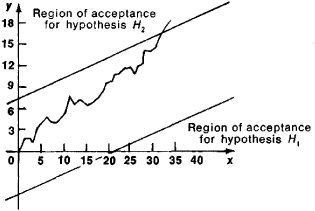Figure 1. Graphical representation of the process of sequential analysis

For example, suppose the problem consists in a choice between the hypotheses H1 and H2 according to the results of independent observations. Hypothesis H1 states that the random variable X has a probability distribution with density f1 (x); hypothesis H2 states that X has density f2 (x). The problem is solved in the following manner. Two numbers A and B are chosen such that 0 < A < B. After the first observation, the ratio λ1 = f2 (x1)/f1 (x1) is computed, where x1 is the result of the first observation. If λ1 < A, then H1 is accepted. If λ1 > B, then H2 is accepted. If A ≤ λ1B, then the process is continued: a second observation is made; the quantity λ2 = f2(x1)f2(x2)/f1(x1)f1(x2), where x2 is the result of the second observation, is analyzed; and appropriate action is taken. The probability is 1 that the process terminates with either the selection of H1 or the selection of H2. The quantities A and B are determined from the condition that the probabilities of errors of the first and second type have the specified values α1 and α2, respectively. An error of the first type is the rejection of hypothesis H1 when it is true, and an error of the second type is the acceptance of H1 when H2 is true.

In practice, it is more convenient to consider instead of λn the logarithms of λn. For example, let hypothesis H1 be that X has a normal distributionwith a= 0 and σ = 1; let hypothesis H2 that X has a normal distribution with a= 0.6 and σ = 1; and let α1 = 0.01 and α2 = 0.03. The corresponding calculations show that in this case A = 1/33, B = 97, and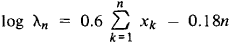Therefore, the inequalities λn < 1/33 and λn > 97 are equivalent to the inequalities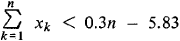and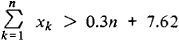respectively. The process of sequential analysis in this case admits of a simple graphic representation (see Figure 1). On the xy-plane there are drawn the two straight lines y= 0.3x —5.83 and y = 0.3x + 7.62 and a broken line with vertices at the points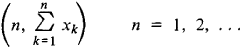If the broken line first leaves the region bounded by the straight lines through the upper boundary, then H2 is accepted. If the broken line leaves the region through the lower boundary, then H1 is accepted. In this example, the method of sequential analysis requires on the average not more than 25 observations to decide between H1 and H2. More than 49 observations would be required to decide between the hypotheses on the basis of samples of a fixed size.

REFERENCES

Blackwell, D., and M. A. Girshick. Teoriia igr i statisticheskikh reshenii. Moscow, 1958. (Translated from English.)
Wald, A. Posledovatel’nyi analiz. Moscow, 1960. (Translated from English.)
Shiriaev, A. N. Statisticheskii posledovatel’nyi analiz. Moscow, 1969.

IU. V. PROKHOROV

References in periodicals archive ?
Franzen, "Fixed length sequential confidence intervals for the probability of response" Sequential Analysis, vol.20, no.1-2, pp.
Sequential analysis. As in Experiment 1, a further ANOVA was conducted with Previous Target x Present Target x Previous Correspondence x Present Correspondence as within-subjects factors.
By leveraging complex sequential analysis technology from Fujitsu Laboratories, the software can detect and analyze warning signs with a high degree of precision.
c) The Observer XT 7.0 executed the lag sequential analysis for the sessions 6, 10 and 13 taking the therapist behaviors Recommendation to group (REC G), Recommendation to P1 (REC P1) and Recommendation to P2 (REC P2) as the criterion codes.
Analizing Interaction: Sequential analysis with SDIS and GSEQ.
Sequential analysis is a set of statistical techniques useful for studying the dynamic nature of behavioral streams, by detecting behavior patterns and uncovering their temporal structure (Quera & Bakeman, 2000).
Sequential analysis methods for examining discussion board content are designed to provide a view of discussion participation not offered by either the statistical metrics or content analysis methods.
Fast Sequential analysis provides new capabilities for flame AA, including semi-quantitative analysis and internal standard corrections.
The methodologies identified were (a) lag sequential analysis, (b) structural analysis, and (c) combined structural and functional analyses.
The serum samples were analyzed sequentially, followed immediately by sequential analysis of the heparin-plasma samples.
This study extends sequential analysis of IRTs to human subjects, investigating how the consequences of response for particular IRT classes affect the distribution of IR[T.sub.n+1], and providing detailed moment-to-moment description of individual response patterns.
The SBCT leadership used the considerations to focus the discussion within a continuous and sequential analysis of the assured mobility fundamentals to successfully tie FM 3-0's see first, understand first, act first, and finish decisively to physical assets owned by the maneuver commander.

Site: Follow: Share:
Open / Close